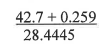# Problem: Calculate the following to the correct number of significant figures. a) 2 b) 1.5 c) 1.51 d) 1.510  e) 1.5103

###### FREE Expert Solution
94% (154 ratings)
###### Problem Details

Calculate the following to the correct number of significant figures.

a) 2

b) 1.5

c) 1.51

d) 1.510

e) 1.5103What scientific concept do you need to know in order to solve this problem?

Our tutors have indicated that to solve this problem you will need to apply the Significant Figures: In Calculations concept. You can view video lessons to learn Significant Figures: In Calculations. Or if you need more Significant Figures: In Calculations practice, you can also practice Significant Figures: In Calculations practice problems.

What is the difficulty of this problem?

Our tutors rated the difficulty ofCalculate the following to the correct number of significant...as medium difficulty.

How long does this problem take to solve?

Our expert Chemistry tutor, Dasha took 1 minute and 59 seconds to solve this problem. You can follow their steps in the video explanation above.

What professor is this problem relevant for?

Based on our data, we think this problem is relevant for Professor Clark's class at OSU.